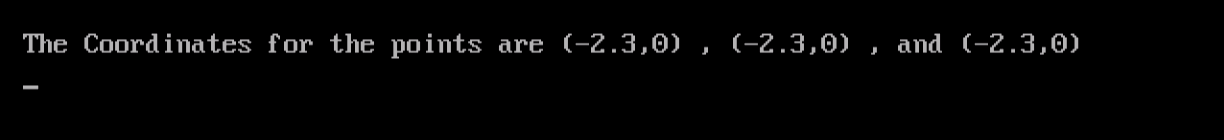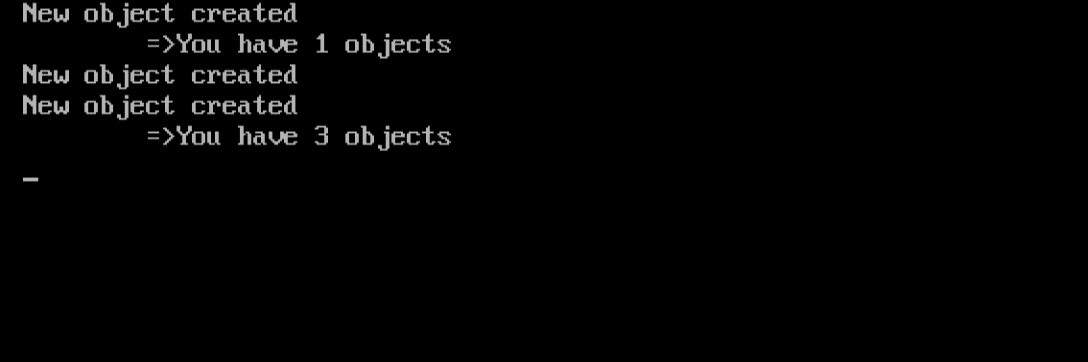System ProgrammingLearn about Destructors and Static Class Attributes in C++

# Learn about Destructors and Static Class Attributes in C++Continuing our discussion on Object-Oriented Programming, let’s finish Constructors, and start talking about their opposite: Destructors.

Initializing Objects using Other Objects
You can initialize an object using another instance (object) of the same class that has been already initialized.

Example
Back to the point class program. In this example, we are going to see how to initialize an object using an already-initialized object of the same class. Consider the following modified version.

```#include <iostream.h>
#include <conio.h>
class point
{
float x,y;
public:
point (float i, float j)
{
x=i;
y=j;
}
void setx(float i)
{ x=i; }
void sety(float j)
{ y=j; }
float getx()
{ return x; }
float gety()
{ return y; }
};
void main()
{
clrscr();
point P1(-2.3,0);
point P2(P1);
point P3 = P1;
cout << "\nThe Coordinates for the points are (" << P1.getx()
<< "," << P1.gety() << ")" << " , (" << P2.getx()
<< "," << P2.gety() << ")" << " , and (" << P3.getx()
<< "," << P3.gety() << ")" << endl;
getch();
}
```

When executed, the program should assign the same set of coordinates to the three objects P1, P2, and P3.First, the object P1 is created and initialized (using the constructor). Next, the object P2 is created and initialized using a copy of P1:

`point P2(P1);`

This will assign the value of each member variable in P1 to its equivalent in P2. This way of initializing an object, using the value of another that belongs to the same class, can be done using another form:

`point P3 = P1;`

The two forms above have the same effect.

Destructors
A Destructor is the opposite of Constructor, and needless to tell you that it has the opposite effect. A constructor is automatically called to initialize a newly-created object. Conversely, a destructor is automatically called when an object is destroyed to de-allocate the memory portion that was allocated to the object on its creation.

A Destructor must:

• Have the same name as its class, but will be preceded with a tilde ~
• Neither have a return value nor arguments.Static Member Variables
Refer back to the point class that we have defined in the above example: it has two member variables x and y. For each instance (object) created of that class, each object will have its own “completely” separate x and y variables. In other words, a portion of memory will be reserved for object P1 that contains 4 bytes segment for the floating-point variable x, and another 4 bytes for the other float variable y. When another object P2 is created, another portion of memory will be allocated for it, with 4 bytes for P2.x and 4 bytes for P2.y. This will take place for each new object instantiated from the point class.

C++ has another type of member variables: the static member variables. Within a class definition, if a variable is declared as static, only one copy of that variable is created for the class, regardless of the number of objects instantiated. Static member variables provide a pretty way to share data between objects of the same class. One common use for static data is to maintain the number of objects created in one class.

Example
The following program uses static member variable to keep track on the number of objects defined.

```#include <conio.h>
#include <iostream.h>
class test
{
static int objcount;
public:
test()
{ 	objcount++;
cout << "New object created\n";
}
int getcount()
{ return objcount; }
};
int test::objcount=0;
void main()
{
clrscr();
test t1;
cout << "\t=>You have " << t1.getcount() << " objects\n";
test t2,t3;
cout << "\t=>You have " << t1.getcount() << " objects\n";
getch();
}
```

Let’s compile and execute this code, and see what we will get:Now, to the explanation:

• To use a static member variable, two distinct statements are needed: one inside the class for the variable declaration:
`static int objcount;`

And, the other outside the class for variable definition:

```int test::objcount=0;
```
• The defined static variable objcount is incremented each time a new object is created. To do this, the following statement is used inside the class constructor:
`objcount++;`
• To get the value of static member variable objcount, the member function getcount() is called:
`t1.getcount()`

In the above statement, the member getcount() that is associated with the object t1 is called. After defining objects t2 and t3, either of the three objects t1, t2, or t3 could be used in the second function call.

`t1.getcount() = t2.getcount() = t3.getcount()`
• One final note regarding the use of the :: operator: it is called the scope resolution operator. This operator is used to specify the class to which a variable or a function belongs. This is useful when defining a member function or a static variable outside the class definition.

Summary
In this article, we have continued our journey with Object-oriented programming by talking about Destructors and Static Member Variables.

• An object can be initialized using another object of the same class. There are two syntax forms to achieve this.
• A Destructor is the opposite of Constructor. It de-allocates memory allocated to an object that is no longer needed.
• A Static member variable is created once for an entire class. It provides a way to share data between objects of the same class.

In the next article, we are going to talk about Strings. An important topic that deserves waiting for. So, see you there.

### How Challenging Is B2B Marketing?

21,501Fans
4,218Followers
103,000Subscribers

### How Challenging Is B2B Marketing?

- Advertisement -